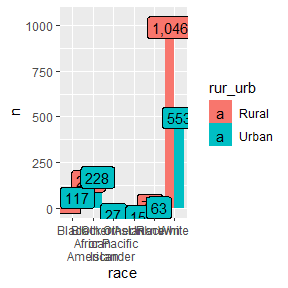This vignette demonstrates how to use the rfars package to download FARS data and use it to compare crash characteristics and trends in rural and urban areas. First we download the data, then make a convenient adjustment to the given coding scheme. Rural and urban crashes and fatalities are then compared on many different dimensions.

## Data

Below we get three years of data for one state, Virginia.

myFARS <- get_fars(years = 2018:2020, states = "VA")

Here we make an adjustment to the standard variable definitions to label all people driving motorcycles as motorcyclists rather than drivers of motorcycles. This makes it easier to refer to motorcyclists later. Note that the myFARS object is a list, with the flat tibble containing the required variables: body_typ (vehicle body type) and per_typ (person type).

myFARS$flat$per_typ <-
ifelse(grepl("motorcycle",
myFARS$flat$body_typ,
ignore.case = TRUE),
"Motorcyclist",
myFARS$flat$per_typ)

The counts() function makes it easy to generate specific types of counts. Here we create a function to run two counts, differentiated by urbanicity, and then stack the results for easy graphing.

compare_counts <- function(myFARS, what, involved=NULL){

bind_rows(
counts(myFARS, what=what, involved=involved, where="rural") %>%
mutate(where = "Rural"),
counts(myFARS, what=what, involved=involved, where="urban") %>%
mutate(where = "Urban")
) %>%
return()

}

## Basic Counts

The number of crashes is a reasonable starting point. Below we use our compare_counts()function and ggplot() to plot the annual count of crashes and fatalities in rural and urban areas, from 2015 to 2020.

compare_counts(myFARS, "crashes") %>%

ggplot(aes(x=date, y=n, label=scales::comma(n))) +
geom_col() +
geom_label(vjust=1) +
facet_wrap(.~where) +
labs(x=NULL, y=NULL, title = "Crashes", fill=NULL)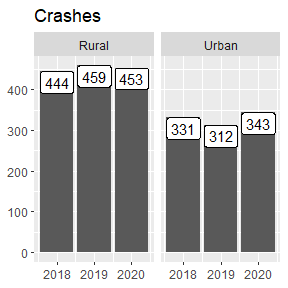compare_counts(myFARS, "fatalities") %>%

ggplot(aes(x=date, y=n, label=scales::comma(n))) +
geom_col() +
geom_label(vjust=1) +
facet_wrap(.~where) +
labs(x=NULL, y=NULL, title = "Fatalities", fill=NULL)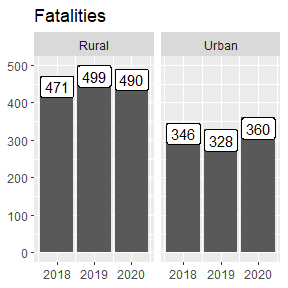## The involved Argument

counts() makes it easy to hone in on specific crash types by using the involved argument. It can be any of: distracted driver, drowsy driver, police pursuit, motorcycle, pedalcyclist, bicyclist, pedestrian, pedbike, young driver, older driver, speeding, alcohol, drugs, hit and run, roadway departure, rollover, or large trucks. Specifying involved will filter the counts to those matching the criterion. For example involved="distracted driver" will return counts associated with crashes involving a distracted driver. Multiple values can be supplied; if so, the resulting counts will satisfy all criteria. That is, they are combined with the and operator (as opposed to or).

Below we loop through all options available in rfars and generate simple plots.

crashfactors <- c("distracted driver", "drowsy driver",
"police pursuit", "motorcycle", "pedalcyclist",
"bicyclist", "pedestrian", "pedbike",
"young driver", "older driver", "speeding",
"alcohol", "drugs", "hit and run",
)

for(crashfactor in crashfactors){

p <-
compare_counts(myFARS, "fatalities", involved = crashfactor) %>%
ggplot(aes(x=year, y=n, label=scales::comma(n))) +
geom_col(position="dodge") +
facet_wrap(.~where) +
geom_label(position = position_dodge(.9), vjust=1) +
labs(title = paste0("Fatalities: ", crashfactor))

print(p)

}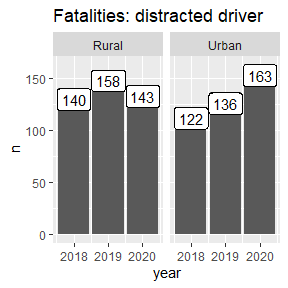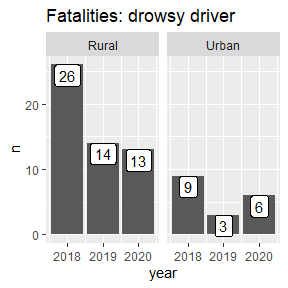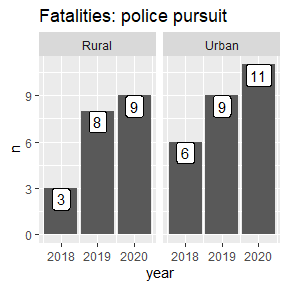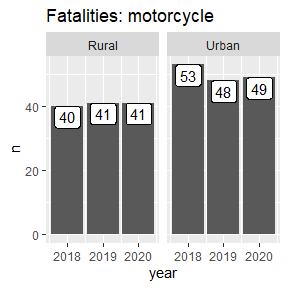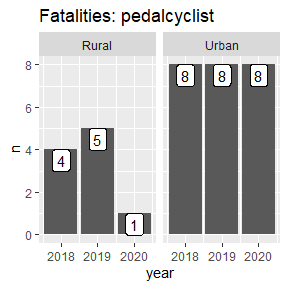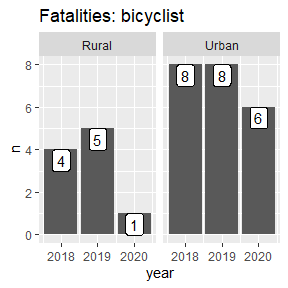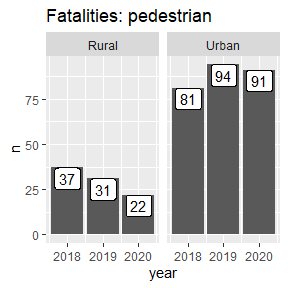#> Note: Young drivers are defined as those between the ages of 15 and 20.
#> Note: Young drivers are defined as those between the ages of 15 and 20.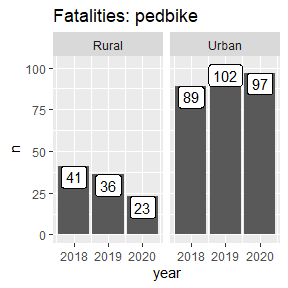#> Note: Older drivers are defined as those aged 65+.
#> Note: Older drivers are defined as those aged 65+.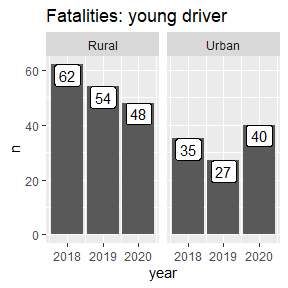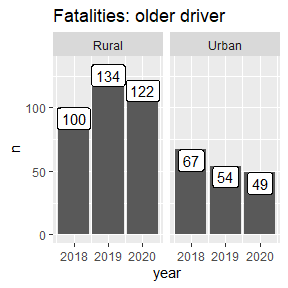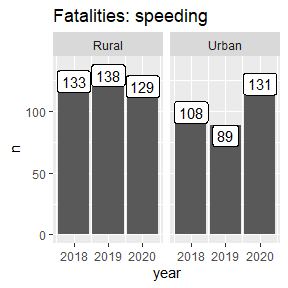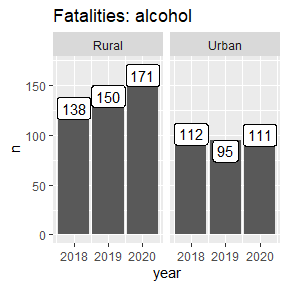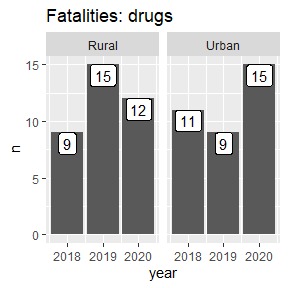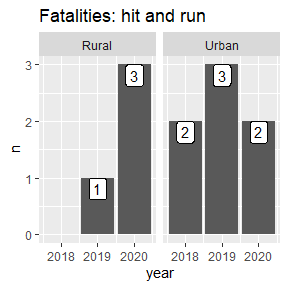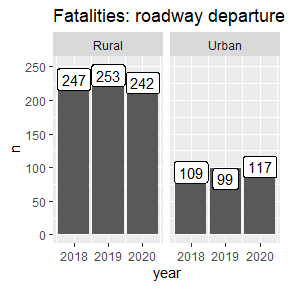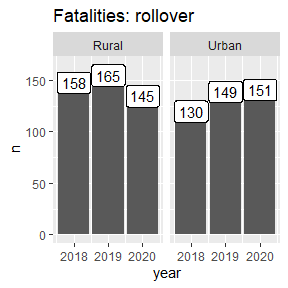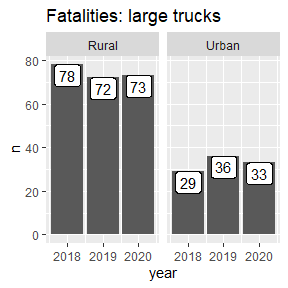## The filterOnly Option

The counts() function has a filterOnly option, which returns pre-summarized data fitting the other specifications (what, where, etc.). This can be useful when generating custom counts. For example, acc_type (Crash Type) is a vehicle-level variable. To count the number of crashes by acc_type, we need to prevent over-counting (as there will be one value for acc_type for each vehicle involved in each crash). Below we take the value associated with veh_no 1 (vehicle number 1). This is reasonable, but may not be appropriate for all analysis situations.

bind_rows(
counts(myFARS,
what = "crashes",
where = "rural",
filterOnly = TRUE
) %>%
filter(veh_no==1) %>% #crash type is on the vehicle-level, this prevents over-counting
select(id, year, acc_type) %>% unique() %>% group_by(acc_type, year) %>% summarize(n=n()) %>%
mutate(where = "Rural"),
counts(myFARS,
what = "crashes",
where = "urban",
filterOnly = TRUE
) %>%
filter(veh_no==1) %>%
select(id, year, acc_type) %>% unique() %>% group_by(acc_type, year) %>% summarize(n=n()) %>%
mutate(where = "Urban")
) %>%
filter(!is.na(acc_type)) %>%
group_by(where, acc_type) %>% summarize(n=sum(n, na.rm=TRUE)) %>%
tidyr::pivot_wider(names_from = "where", values_from = "n") %>%
mutate(Total = Urban + Rural,
rural_pct = Rural/Total) %>%
arrange(desc(Total)) %>%
slice(1:20) %>%
arrange(desc(rural_pct)) %>%
mutate(acc_type = reorder(acc_type, rural_pct)) %>%

ggplot(aes(y=acc_type, x=rural_pct, fill=Rural, label=scales::percent(rural_pct, accuracy = 1))) +
geom_col() +
geom_label(hjust=1, fill="white") +
scale_fill_continuous(labels=scales::comma) +
labs(x=NULL, y=NULL,
title = "20 Most Common Crash Types by Prevalence in Rural Areas") +
theme(plot.title.position = "plot")
#> summarise() has grouped output by 'acc_type'. You can override using the
#> .groups argument.
#> summarise() has grouped output by 'acc_type'. You can override using the
#> .groups argument.
#> summarise() has grouped output by 'where'. You can override using the
#> .groups argument.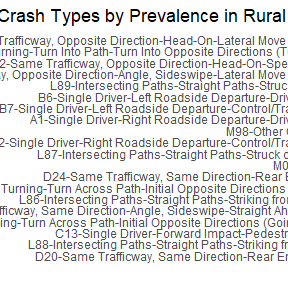## The flat Tibble

It is sometimes easiest to access the data directly, rather than with the counts() function. The object created by use_fars() is a list with five elements, all tibbles: flat, multi_acc, multi_veh, multi_per, and events. The flat data frame contains over 200 variables, and can often provide what’s needed.

Below are several examples:

myFARSflat %>% mutate( vprofile = ifelse(vprofile %in% c("Uphill", "Downhill"), "Up/downhill", vprofile), valign = ifelse(grepl("Curve", valign), "Curve", valign) ) %>% filter(veh_no == 1, #to avoid over-counting rur_urb %in% c("Rural", "Urban"), valign %in% c("Straight", "Curve"), !(vprofile %in% c("Unknown", "Reported as Unknown", "Not Reported")) ) %>% select(id, vprofile, valign, rur_urb) %>% unique() %>% group_by(vprofile, valign, rur_urb) %>% summarize(n = n()) %>% ggplot(aes(x=valign, y=vprofile, fill=n, label=scales::comma(n))) + #geom_tile() + facet_wrap(.~rur_urb) + viridis::scale_fill_viridis() + geom_label() + labs(title = "Roadway Profile and Alignment") #> summarise() has grouped output by 'vprofile', 'valign'. You can override #> using the .groups argument.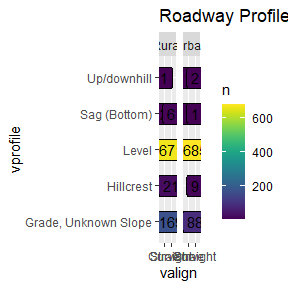myFARSflat %>%
filter(rur_urb %in% c("Rural", "Urban")) %>%
filter(grepl("(K)", inj_sev)) %>%
group_by(rur_urb, per_typ) %>%
summarise(n=n()) %>%
filter(n>2) %>%
mutate(per_typ = stringr::str_wrap(per_typ, 15)) %>%

ggplot(aes(x=per_typ, y=n, fill=rur_urb, label = scales::comma(n))) +
geom_col(position = "dodge") +
geom_label(vjust=1, position = position_dodge(.9)) +
labs(title = "Fatalities by Person Type and Urbanicity")
#> summarise() has grouped output by 'rur_urb'. You can override using the
#> .groups argument.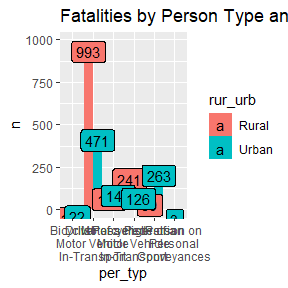myFARS$flat %>% filter(rur_urb %in% c("Rural", "Urban")) %>% filter(grepl("(K)", inj_sev)) %>% group_by(rur_urb, sex) %>% summarise(n=n()) %>% filter(n>90) %>% mutate(sex = stringr::str_wrap(sex, 15)) %>% ggplot(aes(x=sex, y=n, fill=rur_urb, label = scales::comma(n))) + geom_col(position = "dodge") + geom_label(vjust=1, position = position_dodge(.9)) + labs(title = "Fatalities by Sex and Urbanicity") #> summarise() has grouped output by 'rur_urb'. You can override using the #> .groups argument.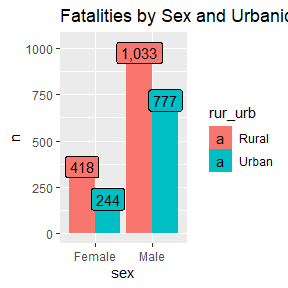myFARS$flat %>%
filter(rur_urb %in% c("Rural", "Urban")) %>%
filter(grepl("(K)", inj_sev)) %>%
group_by(rur_urb, hispanic) %>%
summarise(n=n()) %>%
filter(n>10) %>%
mutate(hispanic = stringr::str_wrap(hispanic, 15)) %>%

ggplot(aes(x=hispanic, y=n, fill=rur_urb, label = scales::comma(n))) +
geom_col(position = "dodge") +
geom_label(vjust=1, position = position_dodge(.9)) +
labs(title = "Fatalities by Ethnicity and Urbanicity")
#> summarise() has grouped output by 'rur_urb'. You can override using the
#> .groups argument.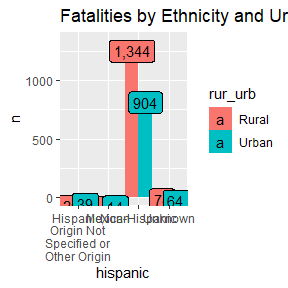myFARS$flat %>% filter(rur_urb %in% c("Rural", "Urban")) %>% filter(grepl("(K)", inj_sev)) %>% filter(!(per_typ %in% c("Bicyclist", "Pedestrian"))) %>% group_by(rur_urb, body_typ) %>% summarise(n=n()) %>% filter(n>30) %>% mutate(body_typ = stringr::str_wrap(body_typ, 80)) %>% ggplot(aes(y=body_typ, x=n, fill=rur_urb, label=scales::comma(n, accuracy = 1))) + geom_col(position = "dodge") + geom_label(hjust=1, position = position_dodge(.9)) + labs(title = "Fatalities by Vehicle Type and Urbanicity") #> summarise() has grouped output by 'rur_urb'. You can override using the #> .groups argument.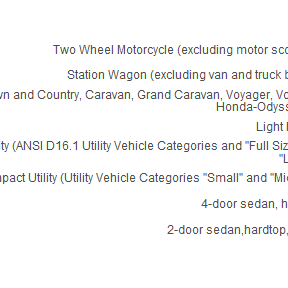myFARS$flat %>%
filter(grepl("(K)", inj_sev),
rur_urb %in% c("Rural", "Urban")) %>%
mutate(age_n = gsub("\\D+","", age) %>% as.numeric()) %>%
group_by(rur_urb, age_n) %>% summarize(n=n()) %>%
filter(age_n <=90) %>%

ggplot(aes(x=age_n, y=n, color = rur_urb)) +
geom_line(size=1.2, alpha=.8) +
labs(title = "Fatalities by Age and Urbanicity")
#> summarise() has grouped output by 'rur_urb'. You can override using the
#> .groups argument.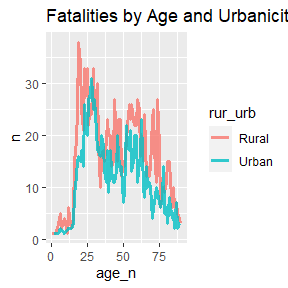myFARS$flat %>% mutate(age_n = gsub("\\D+","", age) %>% as.numeric()) %>% filter(grepl("(K)", inj_sev), rur_urb %in% c("Rural", "Urban"), hour < 25, age_n <= 90) %>% group_by(rur_urb, age_n, hour) %>% summarize(n=n()) %>% ggplot(aes(x=hour, y=age_n, fill=n)) + geom_tile() + facet_wrap(.~rur_urb) + viridis::scale_fill_viridis() + labs(title = "Fatalities by Age, Time of Day, and Urbanicity") #> summarise() has grouped output by 'rur_urb', 'age_n'. You can override using #> the .groups argument.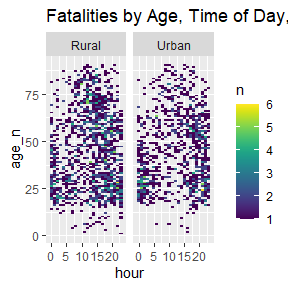## The multi_per Tibble If the flat tibble does not have the required information, it may be in one of the multi_ tibbles. Below, we access the multi_per tibble to visualize fatalities by race. myFARS$multi_per %>%
filter(name == "race") %>%
select(state, st_case, veh_no, per_no, year, race=value) %>%
inner_join(myFARS\$flat) %>%

filter(rur_urb %in% c("Rural", "Urban")) %>%
filter(grepl("(K)", inj_sev)) %>%
group_by(rur_urb, race) %>%
summarise(n=n()) %>%
filter(n>9) %>%
mutate(race = stringr::str_wrap(race, 15)) %>%

ggplot(aes(x=race, y=n, fill=rur_urb, label = scales::comma(n))) +
geom_col(position = "dodge") +
geom_label(vjust=1, position = position_dodge(.9))
#> Joining, by = c("state", "st_case", "veh_no", "per_no", "year")
#> summarise() has grouped output by 'rur_urb'. You can override using the
#> .groups argument.